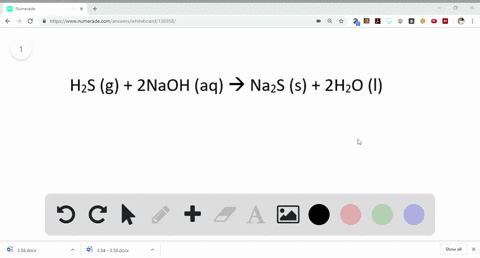Sign up for our free STEM online summer camps starting June 1st!View Summer Courses### When hydrogen sulfide gas is bubbled into a solut…

05:52University of New Mexico

Need more help? Fill out this quick form to get professional live tutoring.

Get live tutoring
Problem 85

Hydrogen sulfide is an impurity in natural gas that must be removed. One common removal method is called the Claus process, which relies on the reaction:
$$8 \mathrm{H}_{2} \mathrm{S}(g)+4 \mathrm{O}_{2}(g) \longrightarrow \mathrm{S}_{8}(l)+8 \mathrm{H}_{2} \mathrm{O}(g)$$
Under optimal conditions the Claus process gives 98$\%$ yield of $S_{8}$ from $\mathrm{H}_{2}$ . If you started with 30.0 g of $\mathrm{H}_{2} \mathrm{S}$ and 50.0 $\mathrm{g}$ of $\mathrm{O}_{2},$ how many grams of $\mathrm{S}_{8}$ would be produced, assuming 98$\%$ yield?

27.66 grams $S_{8}$

## Discussion

You must be signed in to discuss.

## Video Transcript

to solve this question. First, we have to deter mined the Moola months off H two s on order. So the moon or Mars off each to its is 34 grounds for mood. Why, for all to is 32 grounds for mood. We need to deter mined the number off moles in both. It's too. It's on auto. So formula for data mining the number off moles is given weight divided by the molecular mass. So for number off moves for EJ too s will be 30. Divided by 34 equals zero point it it two more on number off. More for or two will be 50. Divided by 32 equals 1.56 works here It's two s will act as a limiting the agent because it was completely consumed in the reaction to form the Louis number off products. As we know that eight mold off H two s produce one moon off essay on the yield given is 98%. So using unitary method, we can deter mine. The SS produced during the reaction which turns out to be zero point 108 months. We know more. The mosque off S ed is 256 so we can determine the amount off essay produce, which is 27.6 rooms, approximately 28 months.